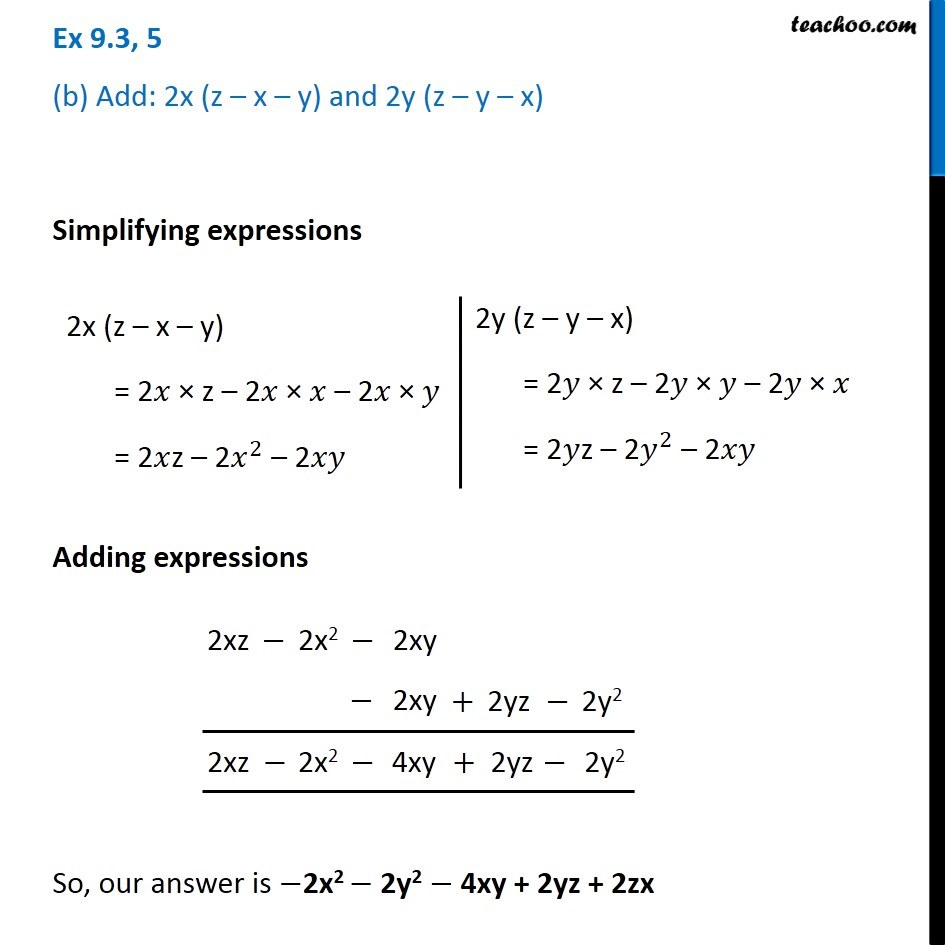Ex 9.3

Chapter 9 Class 8 Algebraic Expressions and Identities
Serial order wiseGet live Maths 1-on-1 Classs - Class 6 to 12

### Transcript

Ex 9.3, 5 (b) Add: 2x (z – x – y) and 2y (z – y – x) Simplifying expressions 2x (z – x – y) = 2𝑥 × z – 2𝑥 × 𝑥 – 2𝑥 × 𝑦 = 2𝑥z – 2𝑥^2 – 2𝑥𝑦 2y (z – y – x) = 2𝑦 × z – 2𝑦 × 𝑦 – 2𝑦 × 𝑥 = 2𝑦z – 2𝑦^2 – 2𝑥𝑦 Adding expressions So, our answer is −2x2 − 2y2 − 4xy + 2yz + 2zx